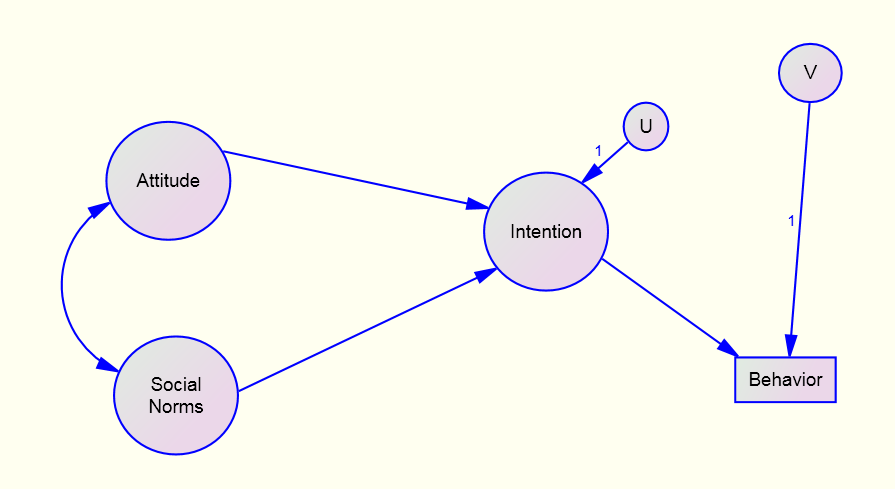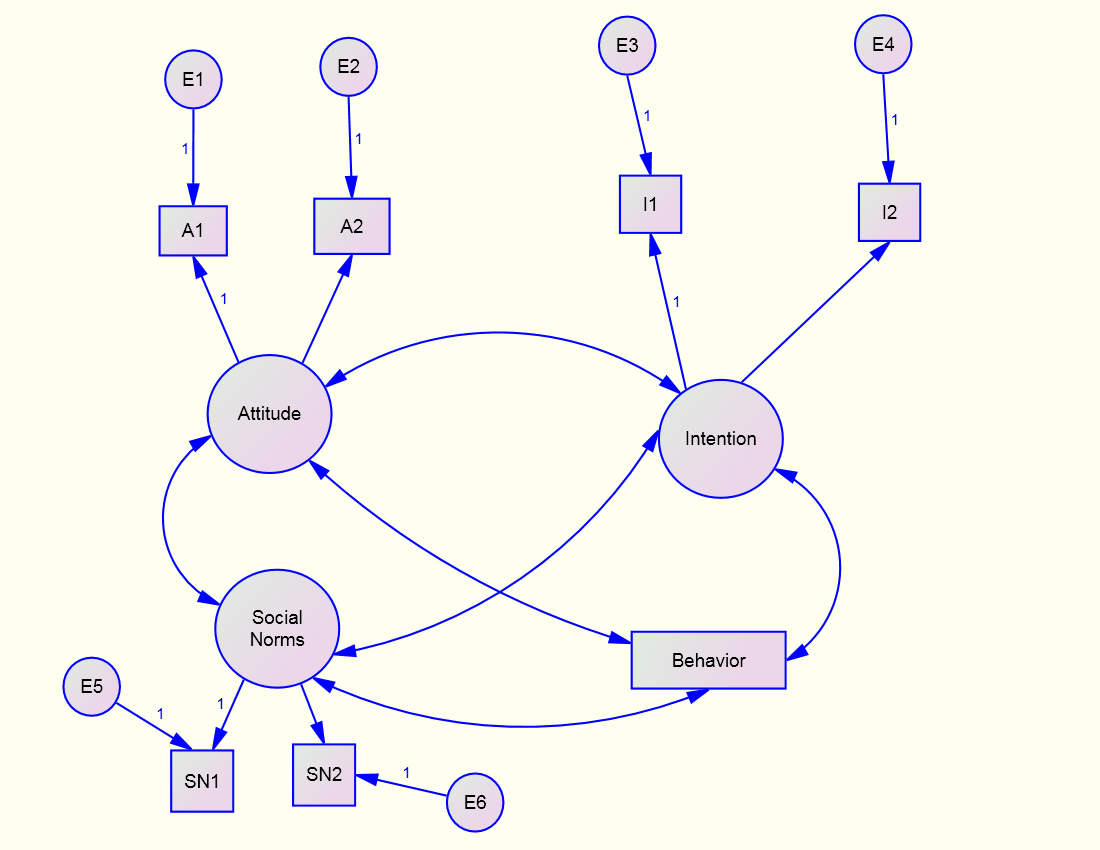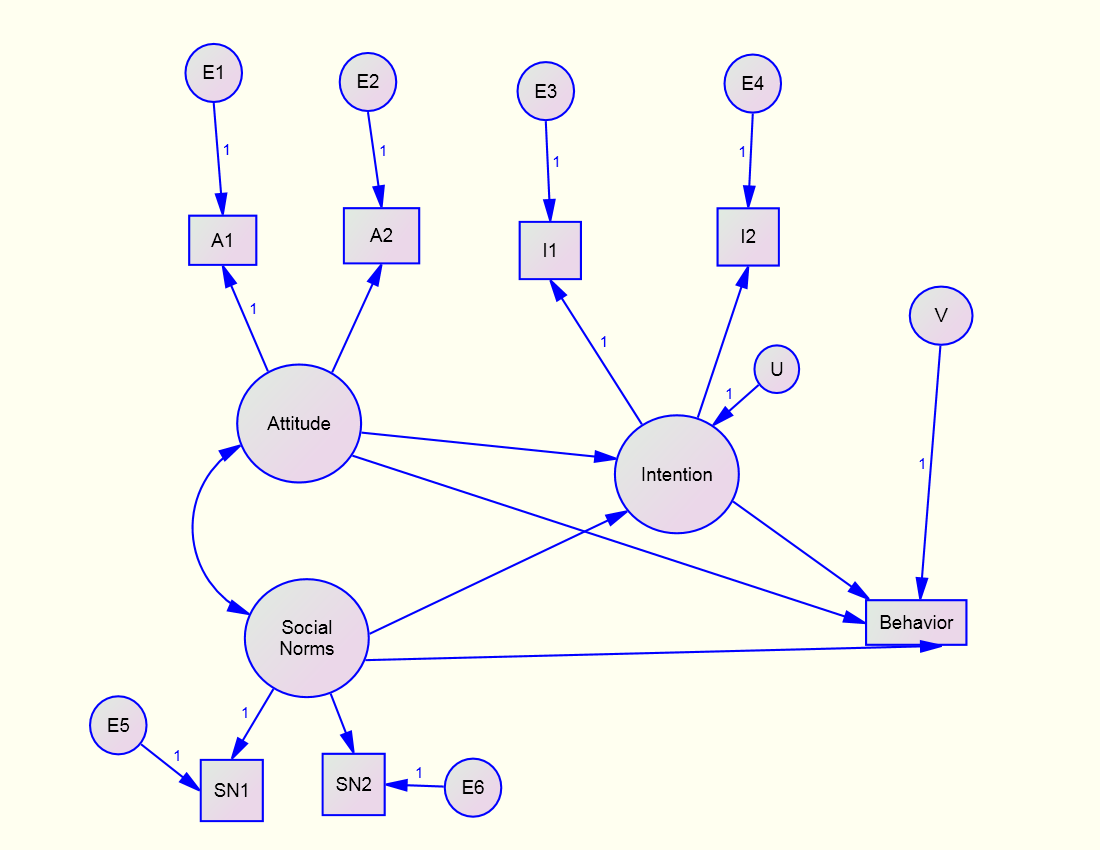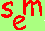David A. Kenny
August 29, 2011Structural Models with Latent Variables

Go to:

Introduction

Measurement Model

Structural Model

Example

Problems with the Wald Test

Williams and Hazer Approach to Measurement Error

Standardization

Missing Data

Introduction

Causal models with latent variables represent a mix of path analysis and confirmatory factor analysis which have been called a hybrid model.  In essence, the measurement model is first estimated and the correlations or covariance matrix between constructs or factors then serves as input to estimate the structural coefficients between constructs or latent variables.  In actuality, both models are simultaneously estimated by a structural equation modeling program such as AMOS, LISREL, or EQS.

Structural Model

·        definition

o   the causal and correlational links between theoretical variables

• constituent parts
• paths
• variances of the exogenous variables
• covariances between exogenous variables
• variances of the disturbances of endogenous variables
• covariances between disturbances
• covariances between disturbances and exogenous variables (usually set to zero)
• test of  specification error
• compare the specified structural model to a model in which the structural model is just-identified

Example (go to Respecification webpage for more details)

Ajzen & Madden (Ajzen, I., & Madden, T. J. (1986). Prediction of goal-directed 'font-size:13.5pt;font-family:"Arial","sans-serif"'>

Model

Measurement Model

Two indicators of Intention, Attitude, and Social Norms

One indicator of Behavior which is assumed to have no measurement error.

Structural Equations

Intention = Attitude + Social Norms +U

Behavior = Intention + V

Parameters

Measurement Model

6 error variances

4 variances of factors

6 covariances between factors (Behavior is considered a factor)

Structural Model

3 paths

1 covariance between exogenous

2 exogenous variances

2 disturbance variances

Degrees of Freedom

Measurement Model

knowns: (8)(7)/2 = 28

unknowns (parameters): 19

df: 9

Structural Model

knowns: (5)(4)/2 = 10

There are just 4 “variables” in the structural model.

unknowns: 8

df: 2

Total Model

df: 2 + 9 = 11

Pure Structural ModelCFA ModelJust-Identified Structural ModelTest the Measurement Model: CFA (or equivalently a Just-identified Structural Model)

Note that to make the structural model just-identified paths must be drawn from Attitude and Social Norms to Behavior

Fit of both models: χ²(11) = 16.09, p = .065

The Ajzen & Madden model has decent fit

Test the Structural Model

Specified Paths

path             estimate         CR           p            χ² diff            p

SN → I:       -0.033         -0.144         .885        0.019     .892

A → I:         0.973         4.116       <.001      16.111   <001

I
B:           0.415         4.653       <.001      23.999  <.001

Problems with Testing of Parameters Using the Wald Test

The Problem (see the Gonzalez & Griffin, Psychological Methods, 2001)

Markers                A I (CR)

A1, I1                    4.116

A2, I1                    4.028

A1, I2                    3.736

A2, I2                    3.670

Result: Critical ratios depend on the choice of the marker. If you change the marker variable, some things change in the model and some things say the same.

What stays the same:

Chi square and the df.

All standard fit indices.

Standardized residuals.
R squared.

What changes:

Critical ratios.

Modification indices.

Solution: Use chi-square difference test if the test is important as it does not depend on the choice of marker.

For the above example it is χ²(1) = 16.111 making the “CR” (the square root of chi square) equal to 4.014.

Williams and Hazer Option to Measurement Error

Overview

A variant of correction for attenuation

Single indicator, not multiple indicators

Must know the reliability of each measure

How

Structural Model: latent variables

Each latent variable causes its measure (path fixed to one)

Each measure has an error path (path fixed to one)

Error variance fixed to

Variance of the measure times one minus the reliability

Of for standardized data, one minus the reliability

Need to test paths using chi square difference test as CR appear to be too conservative.

Fewer variables

Usually smaller standard errors for the paths

Easier to estimate

No Heywood cases

Fewer convergence issues

No test of the measurement model

Assumes the measurement model is correct

Not so traditional and so may meet editorial objections

Standardization (being revised)
Standardization can occur at many places within the modeling process.
the raw data

the data matrix

the model specification

the transformed model

If the model is not standardized, tests refer to the model not the standardized solution.
There are estimation methods based on the assumption that the correlation matrix has been entered (RAMONA), but is rarely used.  This procedure does allow the standardization of latent endogenous variables.
When to Standardize
when the units of measurement not very interpretable

desire to compare coefficients with different units of measurement

more experience with betas than b coefficients

When Not to Standardize

units of measurement are meaningful

paths are usually set equal (so in multiple groups analysis one should analyze the covariance matrix) or paths absolute values compared (e.g., dyadic analysis)

Missing Data (being revised)

Rubin and Little typology

The data are missing at random (MCAR).

Data missing due a variable in the data set which is not missing (MAR).

Data missing due to a variable that is missing or unmeasured (NMAR).

Strategies for handing for missing data – given MAR and MCAR

Data Deletion

Pairwise Deletion – can be problematic

Listwise Deletion

May result in the loss of too many cases

Sample biased: means, variances, & covariances

Imputation – Substitute a Value

Alternatives

Regression

Maximum Likelihood

Multiple Imputation (not used often in SEM)

Full Information Maximum Likelihood (FIML)

By far the most common approach to missing data in SEM

Creates Multiple Groups

Do not get the following output in Amos

Variance-Covariance Matrix of the Measures

Modification Indices

Standardized Residuals

Some Measures of Fit

How to handle an auxiliary variables, i.e., variables in the dataset but not in the model.

Could be important with MAR.

Go to the next SEM page.Go to the main SEM page.